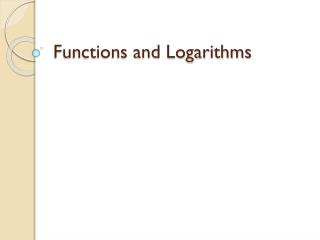DownloadDownload PresentationFunctions and Logarithms

# Functions and Logarithms

Download Presentation## Functions and Logarithms

- - - - - - - - - - - - - - - - - - - - - - - - - - - E N D - - - - - - - - - - - - - - - - - - - - - - - - - - -
##### Presentation Transcript

1. Functions and Logarithms

2. One-to-One Functions • A function f(x) is one-to-one if f(a) ≠ f(b) whenever a ≠ b. • Must pass horizontal line test. Not one-to-one One-to-one

3. Inverses • If a function is one-to-one, then it has an inverse. • Notation: f-1(x) • IMPORTANT:

4. Inverses • To find the inverse of a function, solve for x, “swap” x and y, and solve for y. • Ex: What is the inverse of f(x) = -2x + 4? y = -2x + 4 (“swap” x and y) y – 4 = -2x Therefore,

5. Inverses • Inverse functions are symmetric (reflected) about the line y = x. • Therefore, in order for two functions to be inverses, the results of the composites is x.

6. Inverses • Example: Prove the two functions from our last example are inverses. Now, we must check the other composite! = x Therefore, these two functions must be inverses of one another!!! = x

7. Logarithms • y = logax means ay = x • Ex: log381 = 4 means 34 = 81 • What is the inverse of y = logax? • Since y = logax is ay = x, then the inverse has to be • ax = y

8. Logarithms • Common logarithms: log x means log10x ln x means logex

9. Inverse Properties of Logs (both of these hold true if a > 1 and x > 0) (both of these hold true if x > 0)

10. Inverse Properties of Logs • Example: Solve ln x = 3t + 5 for x. (use each side as an exponent of e) (e and ln are inverses and “undo” each other.)

11. Inverse Property of Logs • Example: Solve e2x = 10 for x. (take the natural log of both sides) (ln and e are inverses and “undo” each other.)

12. Properties of Logarithms • For any real numbers x > 0 and y > 0,

13. Change of Base Property • Since our calculators will not calculate logs of bases other than 10 or e,

14. Example • Sarah invests \$1000 in an account that earns 5.25% interest compounded annually. How long will it take the account to reach \$2500? (divide both sides by 1000) (take a log of both sides…doesn’t matter what base you use!!!) (by my property, exponent comes out front) t ≈ 17.907 years (divide by ln(1.0525))